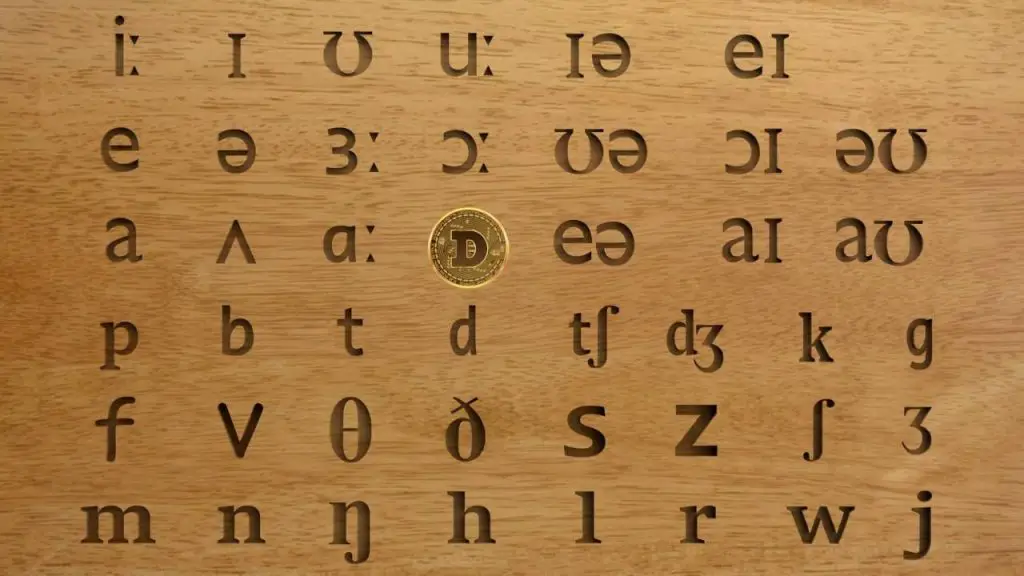# How to Pronounce Dogecoin?Dogecoin is the second most popular altcoin. In this article, we will explain how to pronounce Dogecoin.

Dogecoin pronunciation has two parts — “Doge” and “Coin.” The Doge part is pronounced in the same way as the Dose but with a G sound. The phonetics of Doge is doʊʤ.

We pronounce Doge (doʊʤ) in Dogecoin (doʊʤ kɔɪn) as Dose (doʊs), but we replace the s sound with the ge sound of Age (eɪʤ).

To understand it, let’s first examine how we pronounce Rows. The phonetically we write Rows as roʊz. In this word,

• r stands for R
• oʊ stands for ow
• z stands for s

We pronounce Dose as doʊs. Here,

• d stands for D sound
• oʊ stands for o sound
• s stands for s sound

We also pronounce Rose as roʊz. Here,

• r stands for R sound
• oʊ stands for o sound
• z stands for se sound

We pronounce Age as eɪʤ. Here,

• eɪ stands for A sound
• ʤ stands for ge sound. We use ʤ sound in Dogecoin pronunciation.

In conclusion, we pronounce Doge (doʊʤ) in Dogecoin (doʊʤ kɔɪn) as Dose (doʊs) but we replace the s sound with the ge sound of Age(eɪʤ).